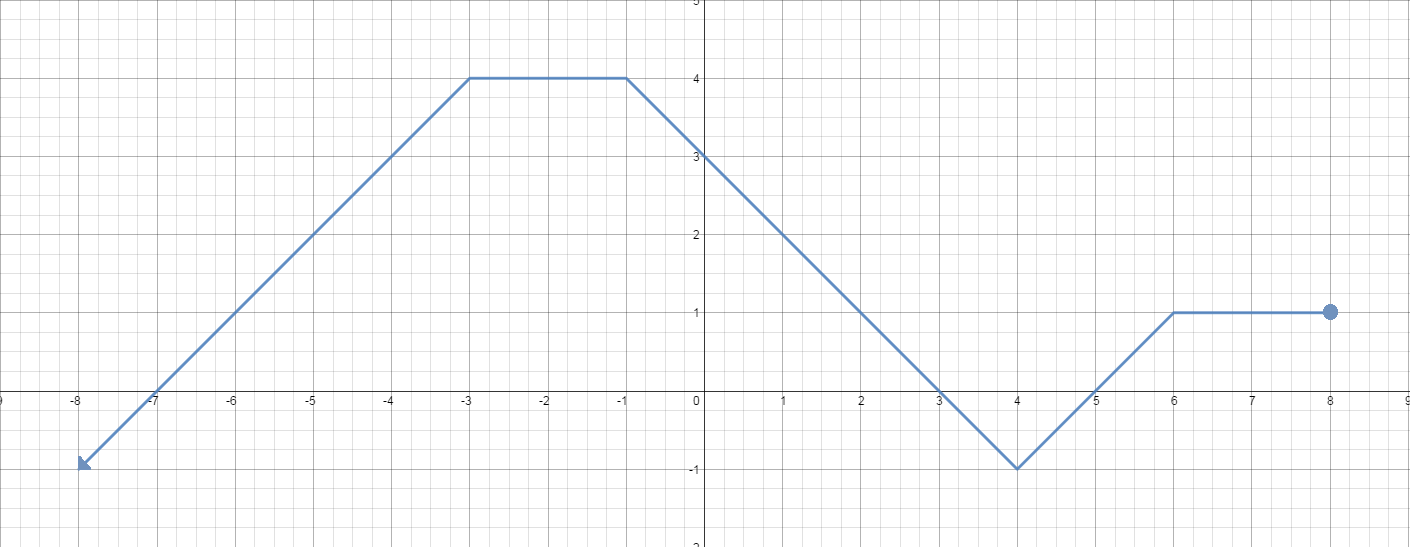# Square root of a function - Radical Functions and Equations

### Square root of a function

What will happen if we put a square root in a function? What will its graph look like? We will tackle these questions in this lesson and see it yourself!

#### Lessons

##### Graphing $y=\sqrt{f(x)}$ from the Graph of $y=f(x)$ Given the graph of $y = f\left( x \right)$ as shown: